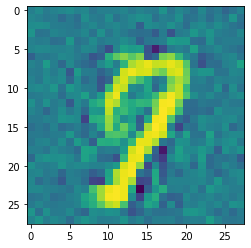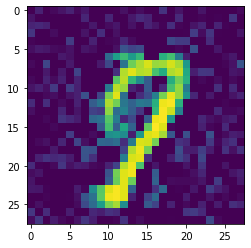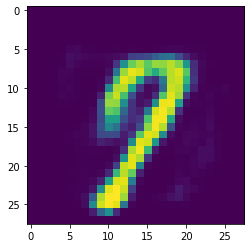# Generative Adversarial Networks: Creating new numbers¶

## Imports¶

:

# importing functions and classes from our framework
from dataset import Dataset
from gan import GAN
from layers import Dense
# other imports
import matplotlib.pyplot as plt
import numpy as np
np.random.seed(333)


## Theory¶

A GAN consists of two neural networks: a generator - the painting forging criminals - and a discriminator - the friend that tries to distinguish whether a painting is real or fake. We will continue by explaining how GANs work by working with the MNIST dataset of handwritten digits. The generator and the discriminator play a minimax game where the generator wants to fool the discriminator and the discriminator wants to detect fakes. This can be expressed in the loss function

$$\underset{G}{\min} \underset{D}{\max} \operatorname{Loss}(D,G) = \mathbb{E}_{x \sim p_{\text{data}}(x)}[\log D(x)] + \mathbb{E}_{z \sim p_{z}(z)}[\log (1- D(G(z)))].$$

Thus we can see that: - the discriminator D tries to label images from the MNIST dataset as real, i.e. learns to assign them a value of 1 - the discriminator D tries to label images that ARE NOT in the MNIST dataset as fake, i.e. learns to assign them a value of 0 - the generator G tries to create images that the discriminator D labels as real, i.e. tries to create images which the discriminator then assigns a value of 1

## Demo¶

Now we load a GAN that has been trained on the MNIST dataset. The generator and the discriminator are multi-layer perceptrons.

:

gan = GAN()
gan.load("mnist_gan") # gan is saved in '/models/mnist_gan'
print(gan)

-------------------- GENERATIVE ADVERSARIAL NETWORK (GAN) --------------------

TOTAL PARAMETERS = 2946577

#################
#   GENERATOR   #
#################

*** 1. Layer: ***
---------------------------------
DENSE 100 -> 256 [LeakyReLU_0.2]
---------------------------------
Total parameters: 25856
---> WEIGHTS: (256, 100)
---> BIASES: (256,)
---------------------------------

*** 2. Layer: ***
---------------------------------
DENSE 256 -> 512 [LeakyReLU_0.2]
---------------------------------
Total parameters: 131584
---> WEIGHTS: (512, 256)
---> BIASES: (512,)
---------------------------------

*** 3. Layer: ***
----------------------------------
DENSE 512 -> 1024 [LeakyReLU_0.2]
----------------------------------
Total parameters: 525312
---> WEIGHTS: (1024, 512)
---> BIASES: (1024,)
----------------------------------

*** 4. Layer: ***
-------------------------
DENSE 1024 -> 784 [Tanh]
-------------------------
Total parameters: 803600
---> WEIGHTS: (784, 1024)
---> BIASES: (784,)
-------------------------

#####################
#   DISCRIMINATOR   #
#####################

*** 1. Layer: ***
----------------------------------
DENSE 784 -> 1024 [LeakyReLU_0.2]
----------------------------------
Total parameters: 803840
---> WEIGHTS: (1024, 784)
---> BIASES: (1024,)
----------------------------------

*** 2. Layer: ***
----------------------------------
DENSE 1024 -> 512 [LeakyReLU_0.2]
----------------------------------
Total parameters: 524800
---> WEIGHTS: (512, 1024)
---> BIASES: (512,)
----------------------------------

*** 3. Layer: ***
---------------------------------
DENSE 512 -> 256 [LeakyReLU_0.2]
---------------------------------
Total parameters: 131328
---> WEIGHTS: (256, 512)
---> BIASES: (256,)
---------------------------------

*** 4. Layer: ***
-------------------------
DENSE 256 -> 1 [Sigmoid]
-------------------------
Total parameters: 257
---> WEIGHTS: (1, 256)
---> BIASES: (1,)
-------------------------

----------------------------------------------------------------------



GANs are, as the name suggests, generative models. Hence training it on handwritten digits, we would expect, that it can come up with new numbers by itself. It is difficult to train GANs (see GANs Lessons) and we haven’t implemented regularizers like dropout or batch normalization. Hence we don’t have optimal results. Training a GAN is a highly unstable optimization problem and it is difficult to find the equilibrium between the discriminator and the generator.

It can be seen that the plain output of the generator looks very noisy. This is partially also caused by the activation function in the output layer, which we chose to be the hyperbolic tangent. Thus the output values are between -1 and 1, but usually we would like grayscale values between 0 and 1 (or between 0 and 255).

:

ganImage = gan.generator.feedforward(gan.sample(1))
plt.imshow(ganImage.reshape(28,28))
plt.show()To achieve proper scaling of the image, i.e. values between 0 and 1, we apply ReLU on the entire image.

:

plt.imshow((ganImage * (ganImage > 0.)).reshape(28,28))
plt.show()In the previous parts of our project, we have seen that denoising autoencoders are very effective in filtering noise out of images. Hence, we will use a denoising autoencoder after our discriminator to generate noise-free digits.

:

from autoencoder import Autoencoder

ae = Autoencoder()
ae.load("ae_gans") # ae is saved in '/models/ae_gans'
print(ae)

-------------------- MULTI LAYER PERCEPTRON (MLP) --------------------

HIDDEN LAYERS = 0
TOTAL PARAMETERS = 79234

*** 1. Layer: ***
-----------------------
DENSE 784 -> 50 [ReLU]
-----------------------
Total parameters: 39250
---> WEIGHTS: (50, 784)
---> BIASES: (50,)
-----------------------

*** 2. Layer: ***
--------------------------
DENSE 50 -> 784 [Sigmoid]
--------------------------
Total parameters: 39984
---> WEIGHTS: (784, 50)
---> BIASES: (784,)
--------------------------

----------------------------------------------------------------------


:

plt.imshow(ae.feedforward(ganImage).reshape(28,28))
plt.show()